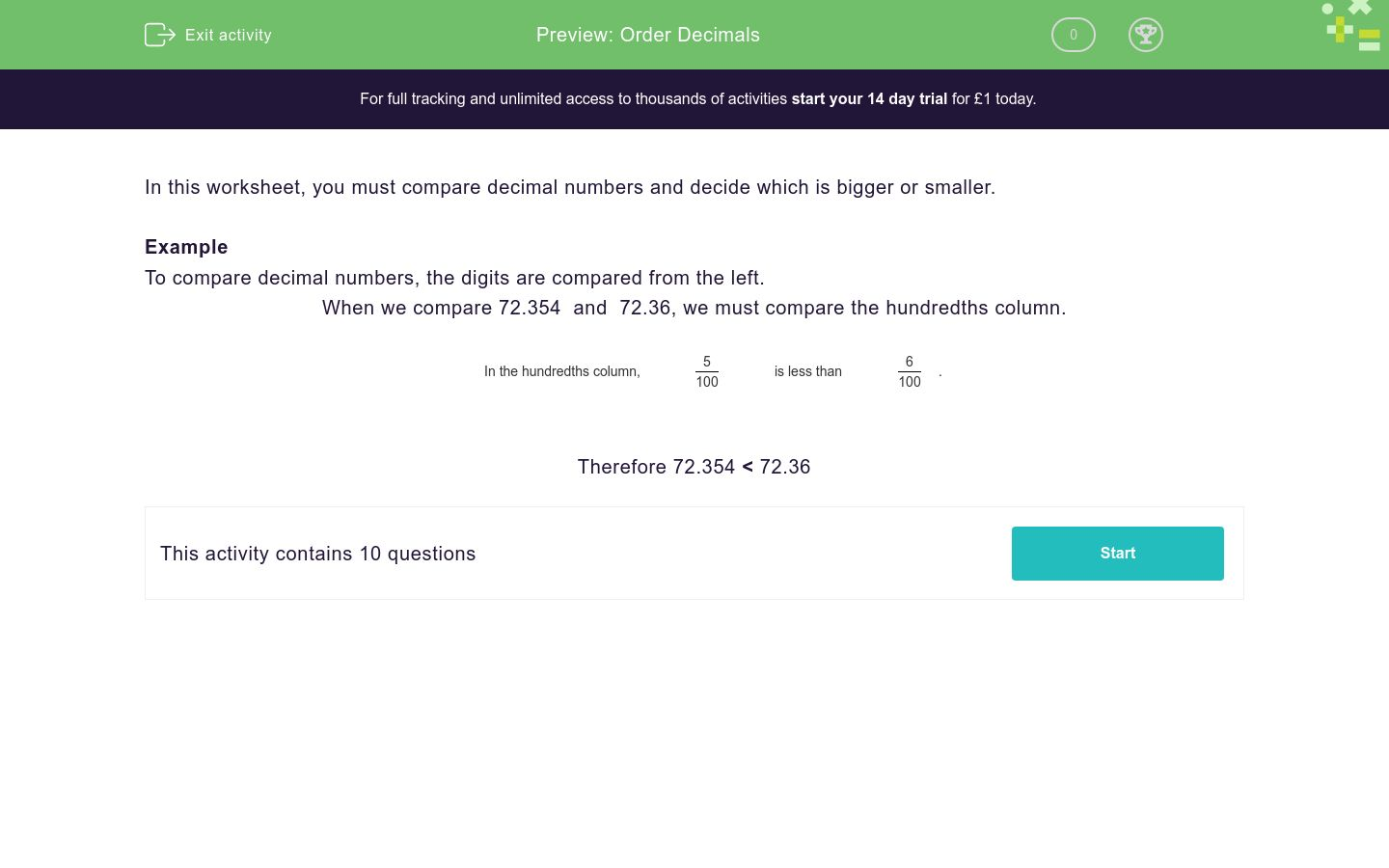# Ordering Decimals

In this worksheet, students decide which of two decimal numbers is bigger or smaller.Key stage:  KS 3

Curriculum topic:   Number

Curriculum subtopic:   Use Place Value for All Numbers

Difficulty level:### QUESTION 1 of 10

In this worksheet, you must compare decimal numbers and decide which is bigger or smaller.

Example

To compare decimal numbers, the digits are compared from the left.

When we compare 72.354  and  72.36, we must compare the hundredths column.

In the hundredths column,
 5 100
is less than
 6 100
.

Therefore 72.354 < 72.36

Insert the symbol < or > between these two numbers.

1.33  _____  1.294

>

<

Insert the symbol < or > between these two numbers.

7.8  _____  7.736

>

<

Insert the symbol < or > between these two numbers.

3.41  _____  3.455

>

<

Insert the symbol < or > between these two numbers.

4.93  _____  4.954

>

<

Insert the symbol < or > between these two numbers.

8.24  _____  8.312

>

<

Insert the symbol < or > between these two numbers.

7.85  _____  7.834

>

<

Insert the symbol < or > between these two numbers.

5.23  _____  5.243

>

<

Insert the symbol < or > between these two numbers.

5.54  _____  4.64

>

<

Insert the symbol < or > between these two numbers.

9.62  _____  9.663

>

<

Insert the symbol < or > between these two numbers.

1.55  _____  1.633

>

<

• Question 1

Insert the symbol < or > between these two numbers.

1.33  _____  1.294

>
• Question 2

Insert the symbol < or > between these two numbers.

7.8  _____  7.736

>
• Question 3

Insert the symbol < or > between these two numbers.

3.41  _____  3.455

<
• Question 4

Insert the symbol < or > between these two numbers.

4.93  _____  4.954

<
• Question 5

Insert the symbol < or > between these two numbers.

8.24  _____  8.312

<
• Question 6

Insert the symbol < or > between these two numbers.

7.85  _____  7.834

>
• Question 7

Insert the symbol < or > between these two numbers.

5.23  _____  5.243

<
• Question 8

Insert the symbol < or > between these two numbers.

5.54  _____  4.64

>
• Question 9

Insert the symbol < or > between these two numbers.

9.62  _____  9.663

<
• Question 10

Insert the symbol < or > between these two numbers.

1.55  _____  1.633

<
---- OR ----

Sign up for a £1 trial so you can track and measure your child's progress on this activity.

### What is EdPlace?

We're your National Curriculum aligned online education content provider helping each child succeed in English, maths and science from year 1 to GCSE. With an EdPlace account you’ll be able to track and measure progress, helping each child achieve their best. We build confidence and attainment by personalising each child’s learning at a level that suits them.

Get started